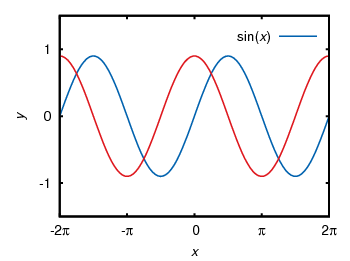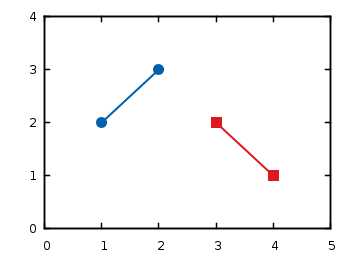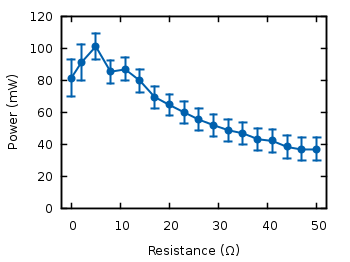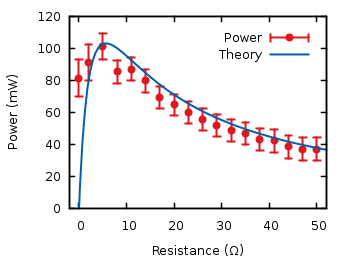# Gnuplotting

Create scientific plots using gnuplot

February 28th, 2011 | No Comments

There is a little update to the epslatex terminal introduction page.

The tics labels can automatically use the math mode from LaTeX by setting the right format option:

set format '$%g$'


Another change is to omit the \resizebox command in LaTeX to include the figure. The problem is that this command also scales your fonts, which you probably don’t want. The alternative is to specify the correct size of your plot before hand and then including the file only with:

\begin{figure}
\input{file}
\end{figure}


April 27th, 2010 | 54 Comments

Gnuplot gives us the opportunity to produce great looking plots in a lot of different formats. Therefore it uses different output terminals that can produce output files or as in the last chapter display the output on your computer screen. In this tutorial we will cover the png, svg, postscript and epslatex terminals. Therefore we will use the same code as in the previous plot functions chapter. We already used the wxt terminal there which displays the result on the screen:

set terminal wxt size 350,262 enhanced font 'Verdana,10' persist


## png, and svg terminal

The png and svg terminals produce more or less the same looking output as the wxt terminal, so you only have to replace the line for the wxt terminal with

set terminal pngcairo size 350,262 enhanced font 'Verdana,10'
set output 'introduction.png'


for png and with

set terminal svg size 350,262 fname 'Verdana' fsize 10
set output 'introduction.svg'


for svg to produce the same figures I have posted in the last chapter. You may have noticed that we use the pngcairo terminal. It exists also a png terminal, but it produces uglier output and doesn’t use the UTF-8 encoding the cairo library does. You may also have noticed that we set the size to a given x,y value. If we don’t do this, the default value of 640,480 is used. The enhanced option tells the terminals that they should interpret something like n_1 for us. But be aware that not all terminals handle the enhanced notation as mentioned in the gnuplot documentation.
Since we want to create an output file in both cases we have to specify one.
The png and svg terminals work very well to produce figures to use on the web as you can see on this page, but for scientific papers or other stuff written in LaTeX we would like to have figures in postscript or pdf format. I always create my pdf files of the plots from the postscript files, so I will cover only the postscript terminals in this introduction.

## postscript terminal

gnuplot has a postscript terminal that can be used to produce figures in the eps format:

set terminal postscript eps enhanced color font 'Helvetica,10'
set output 'introduction.eps'


But this creates very tiny fonts, as stated in the documentation:

In eps mode the whole plot, including the fonts, is reduced to half of the default size.

So if we want to produce the same font and line width dimensions, we have to use this command:

set terminal postscript eps size 3.5,2.62 enhanced color \
font 'Helvetica,20' linewidth 2


It tells the terminal the size in inches of the plot (note that this is the pixel dimension divided by 100) and uses a font size of 20 instead of 10. In addition to that it doubles the given line widths by a factor of 2.

Besides, the postscript terminal can’t handle all UTF-8 input characters and we have to use the enhanced mode to produce greek letter etc. But in opposite to the before mentioned terminals the enhanced mode will work fully for the postscript terminal.

set xlabel '{/Helvetica-Oblique x}'
set ylabel '{/Helvetica-Oblique y}'
set xtics ('-2{/Symbol p}' -2*pi,'-{/Symbol p}' -pi,0, \
'{/Symbol p}' pi,'2{/Symbol p}' 2*pi)
plot f(x) title 'sin({/Helvetica-Oblique x})' with lines linestyle 1, \


Finally, we will get the following figure. Note, that I have converted the resulting eps file to png with Gimp using strong text and graphics antialiasing to show it here. But the overall looking should be the same as with the original eps file.Fig. 1 Sinusoid plotted using the postscript terminal (code to produce this figure)

If we compare Fig. 1 to the original png file made with the pngcairo terminal (Fig. 2) we see that we still have some changes. We need to adjust the tic scales and line widths by hand to get exactly the same result. Note: the font is of course different, because we used another one.Fig. 2 Sinusoid plotted using the pngcairo terminal (code to produce this figure)

This means we should consider if want to create svg and png images or if we want to create eps and png images. In the first case we will use the wxt, svg and pngcairo terminals together.
In the second case we will use only the postscript terminal and create the png files using Gimp or some other image processor.
You may think the handling of symbols e.g. greek letters is very weird in the postscript terminal and you are probably right. But you can also write symbols in LaTeX notation, therefore you have to use the epslatex terminal.

## epslatex terminal

If you have to write formulas or a scientific paper you are mostly interested in postscript/pdf or LaTeX output. The best way is to use both of them. That means to producing the lines etc. in the figure as a postscript image, but generate also a tex file that adds the text for us. The postscript image can be easily converted to a pdf using epstopdf if you needed a pdf version of the image. In order to create the tex file containing our figure labels we will use the epslatex terminal. Basically it has two working modes, a standalone mode that can produce a standalone postscript figure and the normal mode that produces a postscript figure and a tex file to include in your LaTeX document. I think the normal mode is the more common application so I will start with this.

For the normal mode we use the following terminal definition:

set terminal epslatex size 3.5,2.62 color colortext
set output 'introduction.tex'


color and colortext are needed if you have some colored labels, text etc. in your plot. size specifies the size of your plot in inches. You can specify the size alternatively in cm:

set terminal epslatex size 8.89cm,6.65cm color colortext


The easiest way is to specify the size to a value that corresponds with the needed one in your latex document. One way to get these values is to set your view to 100% in your pdf-viewer and measure the size of the area which should contain your figure. In Linux this is easily done using ScreenRuler.

Now we can write the labels etc. in LaTeX notation:

set xlabel '$x$'
set ylabel '$y$'
set format '$%g$'
set xtics ('$-2\pi$' -2*pi,'$-\pi$' -pi,0,'$\pi$' pi,'$2\pi$' 2*pi)
plot f(x) title '$\sin(x)$' with lines linestyle 1, \


Note that it is necessary to use the '' quotes and not "" in order to have interpreted your LaTeX code correctly.

If we run gnuplot on this file, it will generate a introduction.tex and a introduction-inc.eps file. In the LaTeX document we can then include the figure by:

\begin{figure}
\input{introduction}
\end{figure}


Now our figure uses automatically the font and font size of our paper, but we still have to check that the line widths and tics have the size we want to. Also the size of the figure itself has to be correct in order to include it with the \input command without resizing it. Therefore we had to know the size of our figure before hand as mentioned above. Otherwise you should use the \resizebox command in LaTeX, but then it could be that your font size will be incorrect.

\begin{figure}
\resizebox{\columnwidth}{!}{\input{introduction}}
\end{figure}


Note that you need the color package for colored labels. Therefore put \usepackage{color} in the preamble.

If you haven’t any LaTeX document, but want only to produce a figure with LaTeX labels, you can use the standalone mode.
Therefore exchange the above code with

set terminal epslatex size 3.5,2.62 standalone color colortext 10
set output 'introduction.tex'


The last value is the font size of the plot, which you don’t need if you want to include the figure in your LaTeX document.

To produce the standalone figure we have to run more than just the gnuplot command:

$gnuplot introduction.gnu$ latex introduction.tex
$dvips -o introduction.ps introduction.dvi  The first command results in two files: introduction.tex with a LaTeX header and introduction-inc.eps which is the eps file of the figure without any text. The latex command combine the two to a single dvi file and dvips generates a postscript file. Finally I have converted the postscript file with Gimp and we will get this png file:Fig. 3 Sinusoid plotted using the epslatex terminal (code to produce this figure) If we compare this to Fig. 2 it is obvious that we have to fine tune the line widths and tics to get the same result as for the pngcairo terminal. In order to correct it we take the following values: set border linewidth 2 set style line 1 linecolor rgb '#0060ad' linetype 1 linewidth 5 set style line 2 linecolor rgb '#dd181f' linetype 1 linewidth 5 set tics scale 1.25  Applying these settings will finally yield to a figure which looks like it should:Fig. 4 Sinusoid plotted using the epslatex terminal with corrected line width and tics (code to produce this figure) April 27th, 2010 | 12 Comments In this tutorial you will create your first gnuplot figure. Therefore open a text file in your preferred text editor, or directly download the code for this tutorial. Firstly we have to tell gnuplot the output terminal we want to use. The most common are png, svg, postscript or LaTeX output, but first we will start with the output just on the screen using the wxWidgets toolkit. Therefore we have to write this line of code: set terminal wxt size 350,262 enhanced font 'Verdana,10' persist  After that we will specify the style of the output, because the default gnuplot output is ugly in many ways. # Line width of the axes set border linewidth 1.5 # Line styles set style line 1 linecolor rgb '#0060ad' linetype 1 linewidth 2 set style line 2 linecolor rgb '#dd181f' linetype 1 linewidth 2  You can see, a comment starts with a #. We use the set command and specify the border line width and two line styles with the number 1 and 2. For them we specified a colour, the line type where 1 is a normal line and also the line width. In our first graph we want to plot a sinusoid and a cosinus. Therefore we specify our functions and plot them: a = 0.9 f(x) = a * sin(x) g(x) = a * cos(x) # Plot plot f(x) title 'sin(x)' with lines linestyle 1, \ g(x) notitle with lines linestyle 2  As you can see, the definitions of functions in gnuplot are straight forward. We want to plot more than one function that’s why we have to divide the two commands with a comma. The backslash tells gnuplot that we have a line break at this position. Now we save our file as introduction.gnu and execute it by running the following command in BASH under Linux. $ gnuplot introduction.gnu


Under Windows you can start gnuplot with the wgnuplot.exe file and then plot the introduction file by the following command.

gnuplot> load 'path_to_your_file/introduction.gnu'


This should produce in both cases a graph as shown in Fig. 1.

Fig. 1 Our first ugly result (code to produce this figure)

As you can see, we have to tune it a little bit to get a nice graph. Therefore put the following code in front of the function’s definitions (in front of the plot command is sufficient, but I prefer to have the function’s definitions near the plot command).

# Legend
set key at 6.1,1.3
# Axes label
set xlabel 'x'
set ylabel 'y'
# Axes ranges
set xrange [-2*pi:2*pi]
set yrange [-1.5:1.5]
# Axes tics
set xtics ('-2π' -2*pi, '-π' -pi, 0, 'π' pi, '2π' 2*pi)
set ytics 1
set tics scale 0.75


At first we set the legend to a specific position, put labels on the axes and set the ranges of the axes. After that, we specify the positions of the xtics and set strings (note that we can directly use utf-8 strings here) to the given positions. For the ytics we tell gnuplot to make a tic every multiple of 1. Also, we scale the length of the tics, because the default length is a bit too long. Now we can save the file, run the above command again and hopefully get the following figure.

Fig. 2 Our first very nice result (code to produce this figure)

As you have seen in this short tutorial, plotting a function with gnuplot is easy, you only have to tweak a little bit on the appearances of the figure.

Now you can have a look at how to plot data from a data file or how to use other outputs for the figures (png, svg, latex) than your screen.

April 27th, 2010 | 54 Comments

Plotting data like measurement results is probably the most used method of plotting in gnuplot. It works basically like the plotting of functions. But in this case we need a data file and some commands to manipulate the data. First, we will start with the basic plotting of simple data and thereafter look at the plotting of data with errors.

## Simple data

At first we will have a look at a data file. This can be a text file containing the datapoints as columns.

# plotting_data1.dat
# X   Y
1   2
2   3
3   2
4   1


You can plot these by writing

# Set linestyle 1 to blue (#0060ad)
set style line 1 \
linetype 1 linewidth 2 \
pointtype 7 pointsize 1.5

plot 'plotting_data1.dat' with linespoints linestyle 1


Here we also set the point type (pt) and the point size (ps) to use. For the available point styles you can have a look at the ps_symbols file. The resulting plot is presented in Fig. 1.Fig. 1 Plot of the data from plotting_data1.dat (code to produce this figure)

If you have data points that aren’t continuous you can simply tell gnuplot this by inserting one blank line between the data.

# plotting_data2.dat
# X   Y
1   2
2   3

3   2
4   1Fig. 2 Plot of the data from plotting_data2.dat as a non-continuous line (code to produce this figure)

If you want to use another color for the second data and still want to have it in the same file, you can insert a second blank line. You then have to index the data block starting by 0.

# plotting_data3.dat
# First data block (index 0)
# X   Y
1   2
2   3

# Second index block (index 1)
# X   Y
3   2
4   1

# Set first two line styles to blue (#0060ad) and red (#dd181f)
set style line 1 \
linetype 1 linewidth 2 \
pointtype 7 pointsize 1.5
set style line 2 \
linecolor rgb '#dd181f' \
linetype 1 linewidth 2 \
pointtype 5 pointsize 1.5

plot 'plotting_data3.dat' index 0 with linespoints linestyle 1, \
''                   index 1 with linespoints linestyle 2


As you can see, we have added another color and point type and plotted the two datasets by using index and separated the plots by a comma. To reuse the last filename we can just type ''. The result is shown in Fig. 3.Fig. 3 Plot of the data from plotting_data3.dat in two different styles (code to produce this figure)

## Data with errors

Another common task is to plot data with errorbars. Therefore we use the battery.dat file from gnuplots demo files that contains data about the dependence of the power of the battery on the resistance.
Here we want not only to plot the data, but also show the error for the y value (the data is stored in the format: x, y, xerror, yerror).

set xrange [-2:52]
set yrange [0:0.12]
set format y '%.0s'
plot 'battery.dat' using 1:2:4 with yerrorbars linestyle 1, \
''                        with lines linestyle 1


The power values are stored in Watt in the data file, but only has values lower than 1. That’s why we want to use mW as unit. Therefore we set the format option to tell gnuplot to use “mantissa to base of current logscale”, see gnuplot’s documentation. Then in the plot command using tells gnuplot which columns from the data file it should use. Since we want to plot the y errors and the data we need three columns in the first line of the plot command. Using the yerrorbars plotting style it is not possible to combine the points by a line. Therefore we add a second line to the plot command to combine the points with a line. This will give us the resulting Fig. 4.Fig. 4 Plot of the data from battery.dat with y errors (code to produce this figure)

We can avoid the set format command in the last plot by directly manipulating the input data:

set yrange [0:120]
plot 'battery.dat' using 1:($2*1000):($4*1000) with yerrorbars linestyle 1


For achieving this we have to set brackets around the expression and reference the column data with $column_number. In the last plot we will add theoretical data and a legend to the graph: # Legend set key at 50,112 # Theoretical curve P(x) = 1.53**2 * x/(5.67+x)**2 * 1000 plot 'battery.dat' using 1:($2*1000):(\$4*1000) \
title 'Power' with yerrorbars linestyle 2, \
P(x) title 'Theory' with lines linestyle 1


Generally the legend is enabled by the set key command. In addition to that, its position can be specified by set key top left etc. You can also set it directly to one point as we have done it here in order to have enough space between the key and the tics. The title keyword within the plot command specifies the text to be displayed in the legend.Fig. 5 Plot of the data from battery.dat with y errors and a theoretical curve (code to produce this figure)

Now you should be able to plot your own data with gnuplot. You may also want to look at how to plot functions, or dealing with gnuplot’s different output terminals.